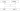# Inheritance

It is possible to acquire all the members of a class and use them in another class by relating the objects of the two classes. This is possible by using inheritance concept. In other words inheritance is deriving new classes from existing classes such that the new classes acquire all the features of existing classes.

## Types Of Inheritance #

There are two types of inheritance which are mentioned below

• Single Inheritance
• Multiple Inheritance

### Single Inheritance #

Producing subclasses from a single super class is called single inheritance. In this there is one super class and one or more subclasses.Single InheritanceIn the above diagram parrot, peacock and sparrow all the three extends the bird which is the example of single inheritance.

### Multiple Inheritance #

Producing subclasses from multiple super classes is called multiple inheritance. In this case there will be more than one super class and there can be one or more subclasses.Multiple InheritanceIn the above diagram class child 1 and class child 2 extends both father and mother which is the example for multiple inheritance.

## ‘Super’ Keyword #

If we create an object to super class, we can access only the super class members, but not the subclass members. But if we create subclass objects, all the members of both super and subclasses are available to it. This is the reason, we always create an object to subclass in inheritance. Sometimes, the super class members and subclass members may have same names. In that case by default, only subclass members are accessible.

``````// by default subclass members are accessible to subclass object
class One {
// super class variable
int i = 10;

// super class method
void show() {
System.out.println("super class method:i=" + i);
}
}

class Two extends One {
// subclass variable
int i = 20;

//subclass method
void show() {
System.out.println("subclass method:i=" + i);
}
}

class Super1 {
public static void main(String args[]) {
// create subclass object
Two t = new Two();
// this will call subclass method only
t.show();
}
}
``````

The output is as follows

``````subclass method:i= 20
``````

Here we observe that a call to subclass method, `t.show()`; calls and executes only subclass method. And hence the subclass instance variable `i` value 20 is displayed. In such case, how to access the super class members from subclass is the question. For, this purpose, super keyword is invented.

``````// super - to access the super class method and variable
class One {
// super class variable
int i = 10;

// super class method
void show() {
System.out.println("super class method:i=" + i);
}
}

class Two extends One {
// subclass variable
int i = 20;

//subclass method
void show() {
System.out.println("subclass method:i=" + i);
// using super to call super class method
super.show();
// using super to access super class variable
System.out.println("super i =" + super.i);
}
}

class Super1 {
public static void main(String args[]) {
// create subclass object
Two t = new Two();
// this will call subclass method only
t.show();
}
}
``````

The output is as follows

``````subclass method:i= 20
super class mmethod:i= 10
super i= 10
``````

In the above program we can access the super class method and instance variable by using super keyword from subclass.